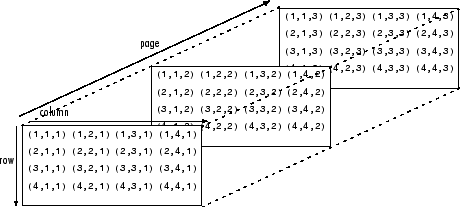# pagenorm

Page-wise matrix or vector norm

## Syntax

``N = pagenorm(X)``
``N = pagenorm(X,p)``
``N = pagenorm(X,"fro")``
``N = pagenorm(V)``
``N = pagenorm(V,p)``

## Description

example

````N = pagenorm(X)` returns the 2-norm of each matrix page of N-D array `X`. Each page of the output array `N` is given by ```N(1,1,i) = norm(X(:,:,i))```.If `X` has more than three dimensions, then `pagenorm` returns an N-D array with the same dimensions, as in `N(1,1,i,j,k) = norm(X(:,:,i,j,k))`.```

example

````N = pagenorm(X,p)` returns the p-norm of each page of `X`, where `p` is `1`, `2`, or `Inf`. If `p = 1`, then `N` contains the maximum absolute column sum of each page of `X`.If `p = 2`, then `N` contains the maximum singular value of each page of `X`.If `p = Inf`, then `N` contains the maximum absolute row sum of each page of `X`. ```

example

````N = pagenorm(X,"fro")` returns the Frobenius norm of each page of `X`.```

example

````N = pagenorm(V)`, where `V` is an array with a vector on each page, returns the 2-norm of each page of N-D array `V`. Each page of the output array `N` is given by `N(1,1,i) = norm(V(:,1,i))` or ```N(1,1,i) = norm(V(1,:,i))```, depending on the orientation of the vectors.```

example

````N = pagenorm(V,p)` returns the generalized vector p-norm of each page of `V`, where `p` is any positive real value or `Inf`.```

## Examples

collapse all

Create a 3-D array with a matrix on each of two pages.

```a = magic(3); b = pascal(3); A = cat(3,a,b);```

Calculate the 2-norm of each page. This command returns the maximum singular value of each matrix.

`N = pagenorm(A)`
```N = N(:,:,1) = 15 N(:,:,2) = 7.8730 ```

Now calculate the infinity norm of each page. This command returns the maximum absolute row sum of each matrix.

`Ninf = pagenorm(A,Inf)`
```Ninf = Ninf(:,:,1) = 15 Ninf(:,:,2) = 10 ```

When each page of a multidimensional array contains a vector, you can use `pagenorm` to calculate vector norms of each page.

Create a 3-D array with a vector on each of three pages.

```a = 1:10; b = 11:20; c = 21:30; V = cat(3,a,b,c);```

Calculate the 2-norm of each page in the array. This command returns the magnitude of each vector.

`N = pagenorm(V)`
```N = N(:,:,1) = 19.6214 N(:,:,2) = 49.8498 N(:,:,3) = 81.1480 ```

Now calculate the 1-norm of each page. This command returns the sum of the element magnitudes for each vector.

`N1 = pagenorm(V,1)`
```N1 = N1(:,:,1) = 55 N1(:,:,2) = 155 N1(:,:,3) = 255 ```

Create a 3-D array with a matrix on each of three pages.

```a = randi(10,3,3); b = hilb(3); c = pascal(3); A = cat(3,a,b,c);```

Calculate the Frobenius norm of each page.

`N = pagenorm(A,"fro")`
```N = N(:,:,1) = 21.9089 N(:,:,2) = 1.4136 N(:,:,3) = 7.9373 ```

## Input Arguments

collapse all

Input array with matrix pages, specified as a matrix or multidimensional array.

Data Types: `single` | `double`
Complex Number Support: Yes

Input array with vector pages, specified as a vector or multidimensional array.

Data Types: `single` | `double`
Complex Number Support: Yes

Norm type, specified as `2` (default), a positive real scalar, or `Inf`. The valid values of `p` and what they return depend on whether each page of the input array is a matrix or vector, as shown in the table. See `norm` for definitions of each norm type.

Note

This table does not reflect the actual algorithms used in calculations.

pMatrix pagesVector pages
`1``max(sum(abs(A)))``sum(abs(u))`
`2` `max(svd(A))``sum(abs(u).^2)^(1/2)`
Positive, real-valued numeric scalar`sum(abs(u).^p)^(1/p)`
`Inf``max(sum(abs(A')))``max(abs(u))`

Example: `pagenorm(X,Inf)` returns the maximum absolute row sum of each matrix page in `X`.

Example: `pagenorm(V,2)` returns the magnitude of each vector page in `V`.

## Output Arguments

collapse all

Norm value, returned as a scalar or multidimensional array. The norm gives a measure of the magnitude of the elements. By convention, `pagenorm` returns `NaN` if the input contains any `NaN` values on a page.

collapse all

### Array Pages

Page-wise functions like `pagenorm` operate on 2-D matrices that have been arranged into a multidimensional array. For example, with a 3-D array the elements in the third dimension of the array are commonly called pages because they stack on top of each other like pages in a book. Each page is a matrix that gets operated on by the function.You can also assemble a collection of 2-D matrices into a higher dimensional array, like a 4-D or 5-D array, and in these cases `pagenorm` still treats the fundamental unit of the array as a 2-D matrix that gets operated on, such as `X(:,:,i,j,k,l)`.

The `cat` function is useful to assemble a collection of matrices into a multidimensional array, and the `zeros` function is useful to preallocate a multidimensional array.

## Tips

• The `squeeze` function is useful to reshape results from `pagenorm` as a column vector.

• When `pagenorm` operates on a single matrix or vector, the results might differ from `norm` slightly due to floating-point round-off error.

## Version History

Introduced in R2022b